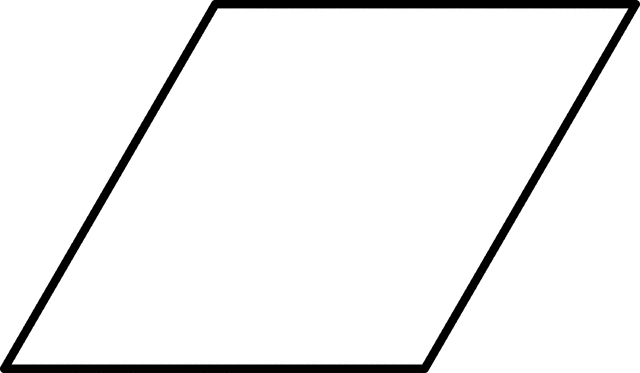# Program for Circumference of a Parallelogram

Given the sides of Parallelogram then calculate the circumference.

Examples :

```Input: a = 10, b = 8
Output: 36.00

Input: a = 25.12, b = 20.4
Output: 91.04
```

## Recommended: Please try your approach on {IDE} first, before moving on to the solution.

The opposite sides of a parallelogram are of equal length and parallel. The angles are pairwise equal but not necessarily 90 degree. The circumference of a Parallelogram can be computed as sum of two adjacent sides each multiplied by 2.Formula used to calculate circumference of a Parallelogram:
(2*a)+(2*b)

## C++

 `// C Program to calculate the ` `// Circumference of a Parallelogram. ` `#include ` ` `  `float` `circumferenceparallelogram(``float` `a, ``float` `b) ` `{ ` `    ``return` `((2 * a) + (2 * b)); ` `} ` ` `  `int` `main() ` `{ ` `    ``float` `a = 10, b = 8; ` `    ``printf``(``"Circumference of a given Parallelogram is : %.2f"``, ` `           ``circumferenceparallelogram(a, b)); ` `    ``return` `0; ` `} `

## Java

 `// Java Program To Calculate ` `// Circumference of a Parallelogram ` `import` `java.io.*; ` ` `  `class` `GFG ` `{ ` `    ``static` `float` `circumferenceparallelogram(``float` `a, ``float` `b) ` `    ``{ ` `    ``return` `((``2` `* a) + (``2` `* b)); ` `    ``} ` ` `  `    ``// Driver code ` `    ``public` `static` `void` `main(String arg[]) ` `    ``{ ` `        ``float` `a = ``10``, b = ``8``; ` `        ``System.out.print(``"Circumference of a given Parallelogram is :"``); ` `        ``System.out.println(circumferenceparallelogram(a, b)); ` `    ``} ` `} ` ` `  `// This code is contributed by Anant Agarwal. `

## Python3

 `# Python3 Program to calculate the ` `# Circumference of a Parallelogram ` ` `  `# Utility Function ` `def` `circumferenceparallelogram(a,b): ` `    ``return` `((``2` `*` `a) ``+` `(``2` `*` `b)) ` ` `  `# Driver Function ` `a ``=` `10` `b ``=` `8` ` `  `print``(``"Circumference of a given Parallelogram is :"``, ` `         ``round``(circumferenceparallelogram(a, b),``4``)) ` ` `  `# This code is contributed ` `# by Azkia Anam. `

## C#

 `// C# Program To Calculate ` `// Circumference of a Parallelogram ` `using` `System; ` ` `  `class` `GFG ` `{ ` `    ``static` `float` `circumferenceparallelogram(``float` `a, ` `                                            ``float` `b) ` `    ``{ ` `    ``return` `((2 * a) + (2 * b)); ` `    ``} ` ` `  `    ``// Driver code ` `    ``public` `static` `void` `Main() ` `    ``{ ` `        ``float` `a = 10, b = 8; ` `        ``Console.Write(``"Circumference of a"` `+  ` `                       ``"given Parallelogram is :"``); ` `                        `  `        ``Console.Write(circumferenceparallelogram(a, b)); ` `    ``} ` `} ` ` `  `// This code is contributed by vt_m. `

## PHP

 ` `

Output :

```Circumference of a given parallelogram is : 36.00
```

Attention reader! Don’t stop learning now. Get hold of all the important DSA concepts with the DSA Self Paced Course at a student-friendly price and become industry ready.

My Personal Notes arrow_drop_upCheck out this Author's contributed articles.

If you like GeeksforGeeks and would like to contribute, you can also write an article using contribute.geeksforgeeks.org or mail your article to contribute@geeksforgeeks.org. See your article appearing on the GeeksforGeeks main page and help other Geeks.

Please Improve this article if you find anything incorrect by clicking on the "Improve Article" button below.

Improved By : vt_m

Article Tags :
Practice Tags :

Be the First to upvote.

Please write to us at contribute@geeksforgeeks.org to report any issue with the above content.